# NCERT Exemplar Class 10 Science Solutions for Chapter 5 - Periodic Classification of Elements

## NCERT Exemplar Solutions Class 10 Science Chapter 5 – Free PDF Download

The NCERT Exemplar Class 10 Science Chapter 5 Periodic Classification of Elements helps you in studying all important concepts in the chapter from the exam point of view. NCERT Exemplar Solution covers concepts that are more likely to be asked in examinations. Hence, students are advised to go through the NCERT Exemplar to ace their examinations. In Chapter 5, students learn the basics of the classification of elements, a bit of history about the periodic table and the position of the elements in the modern periodic table.

They will further recall Dobereiner‘s law of triads and Newlands law of octaves and also interpret Mendeleev’s periodic table. While learning about these different laws can be confusing, we are offering free NCERT Exemplar for Class 10 Science Chapter 5 – Periodic Classification of Elements here to further help students in getting familiar with and recall all the concepts properly. The exemplars contain detailed answers prepared by the subject experts and will enable students to study effectively, as well as to be ready to tackle any question appearing in the board exam.

Have a glimpse of the Class 10 Science Chapter 5 NCERT Exemplar PDF below.

### Download the PDF of the NCERT Exemplar for Class 10 Science Chapter 5 – Periodic Classification of Elements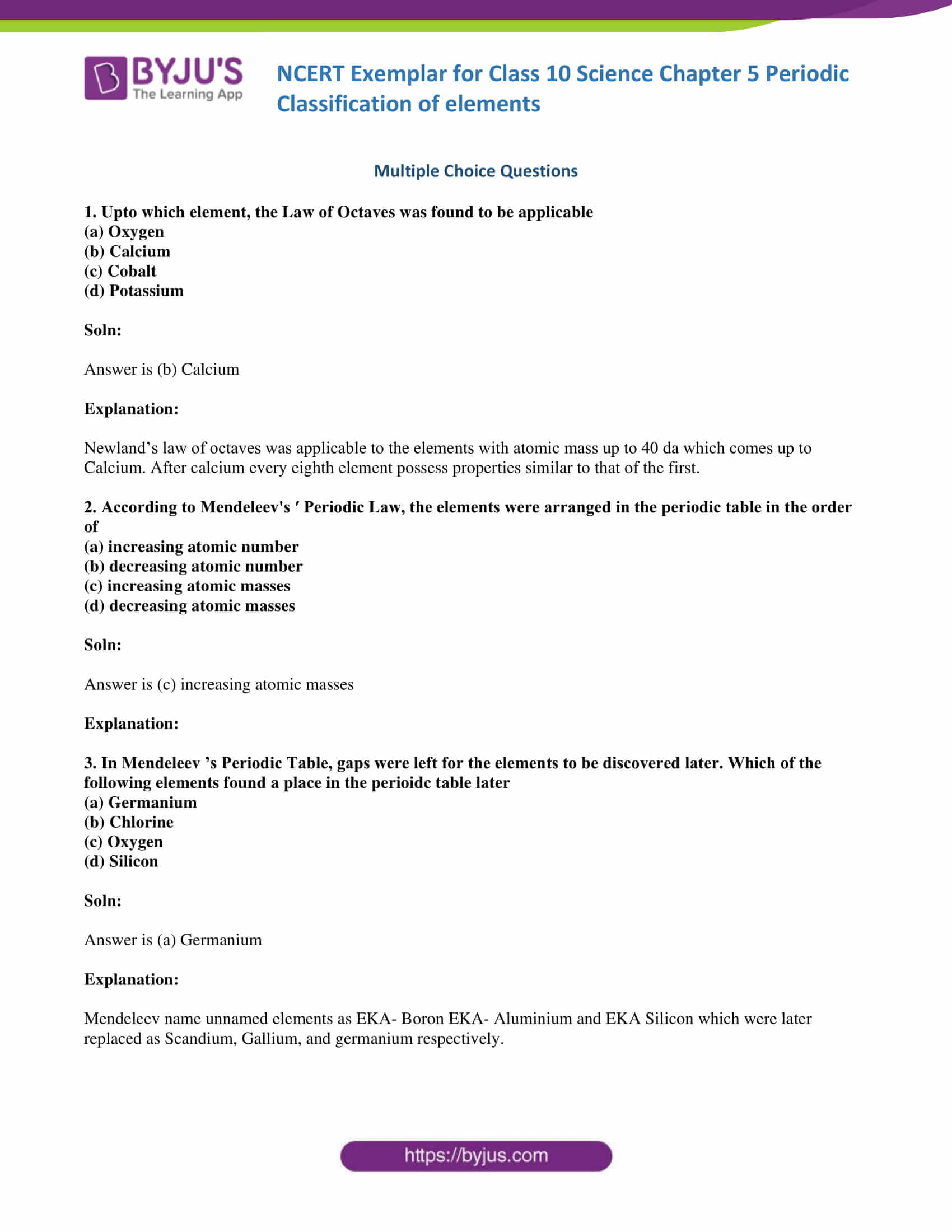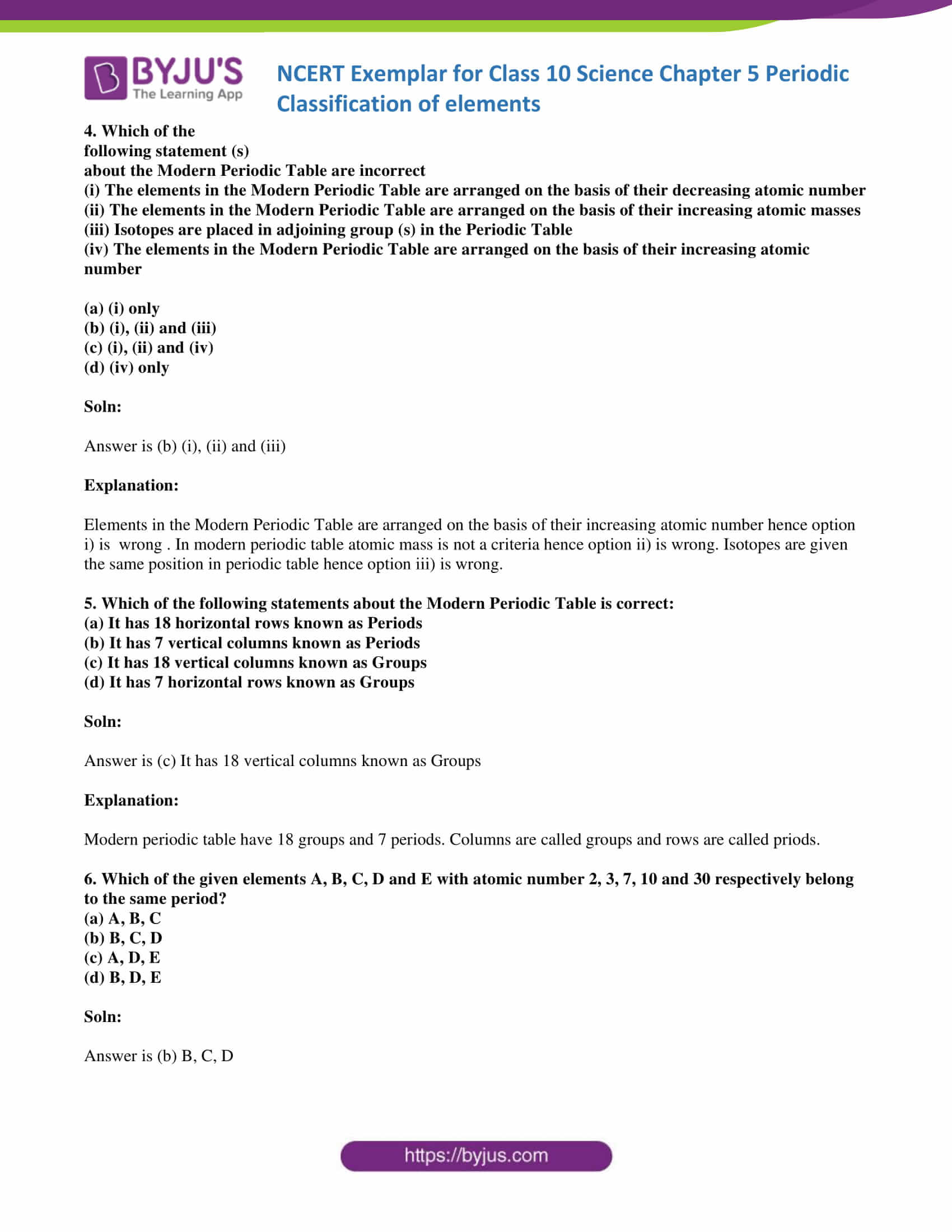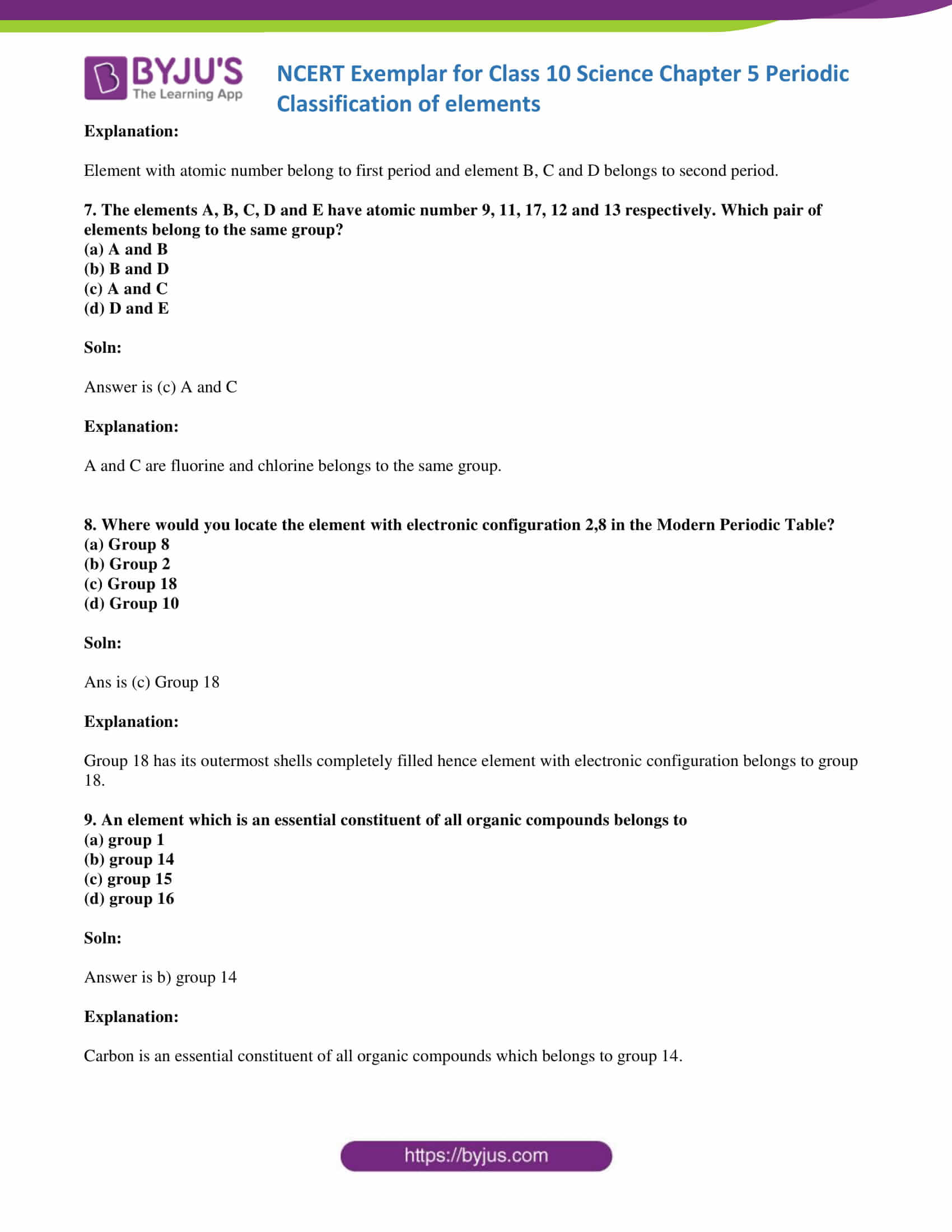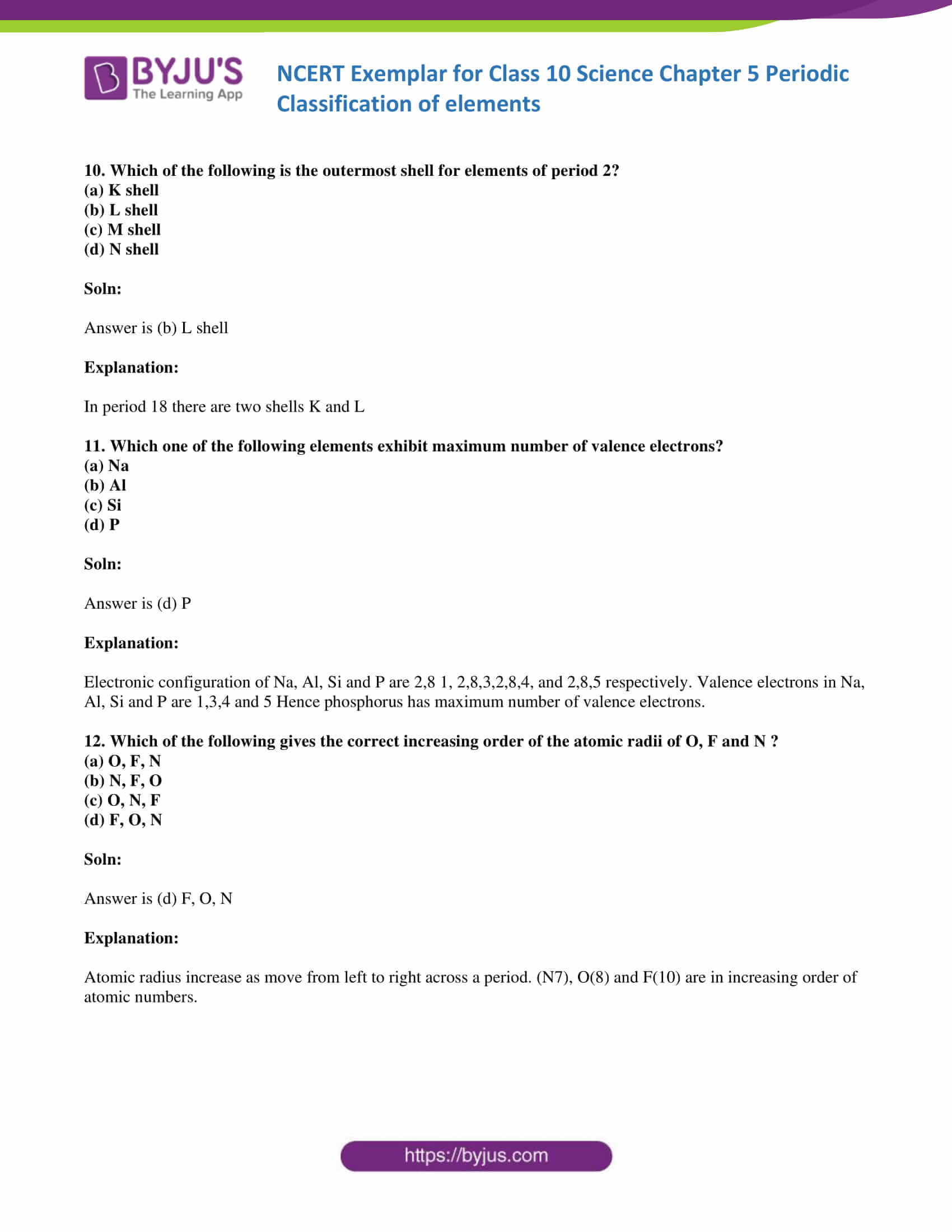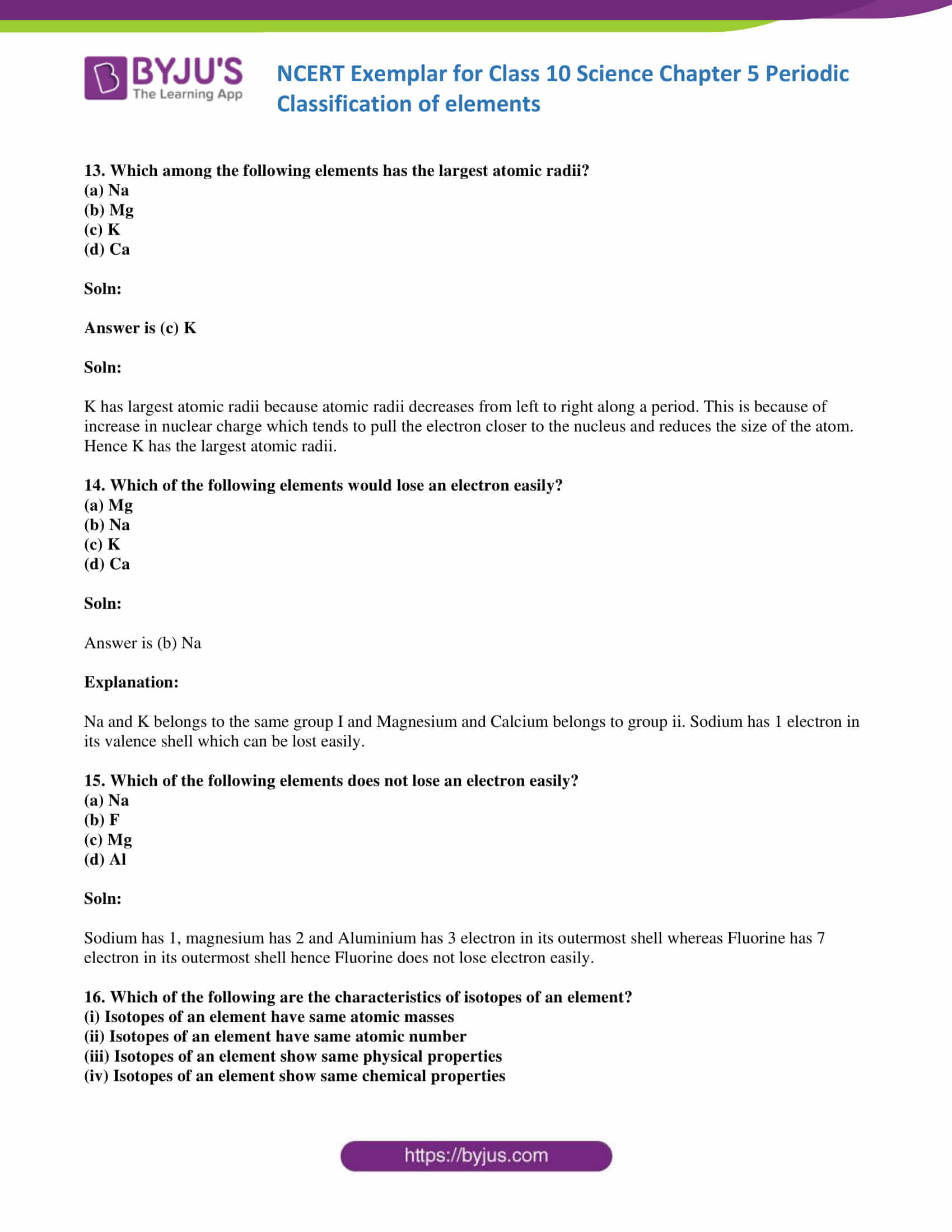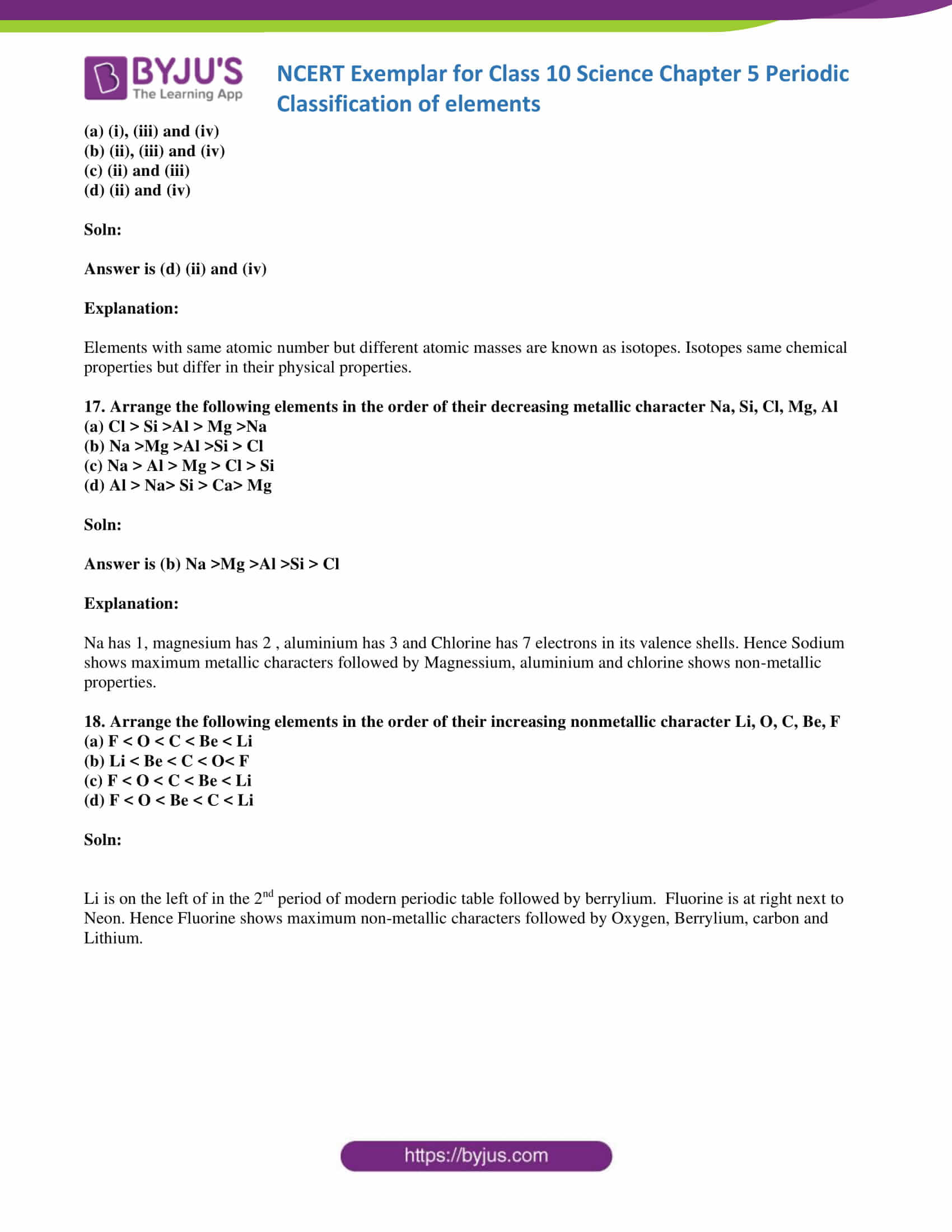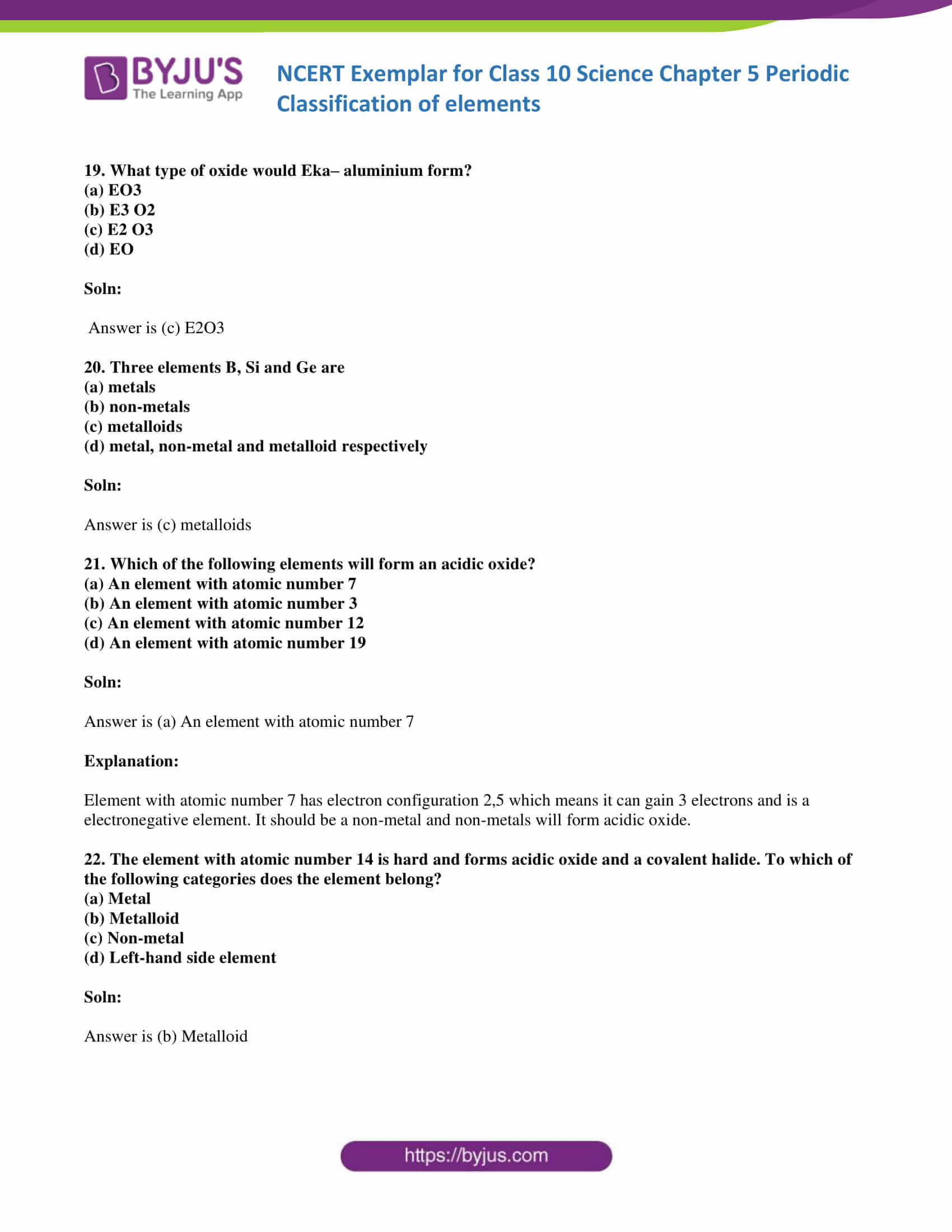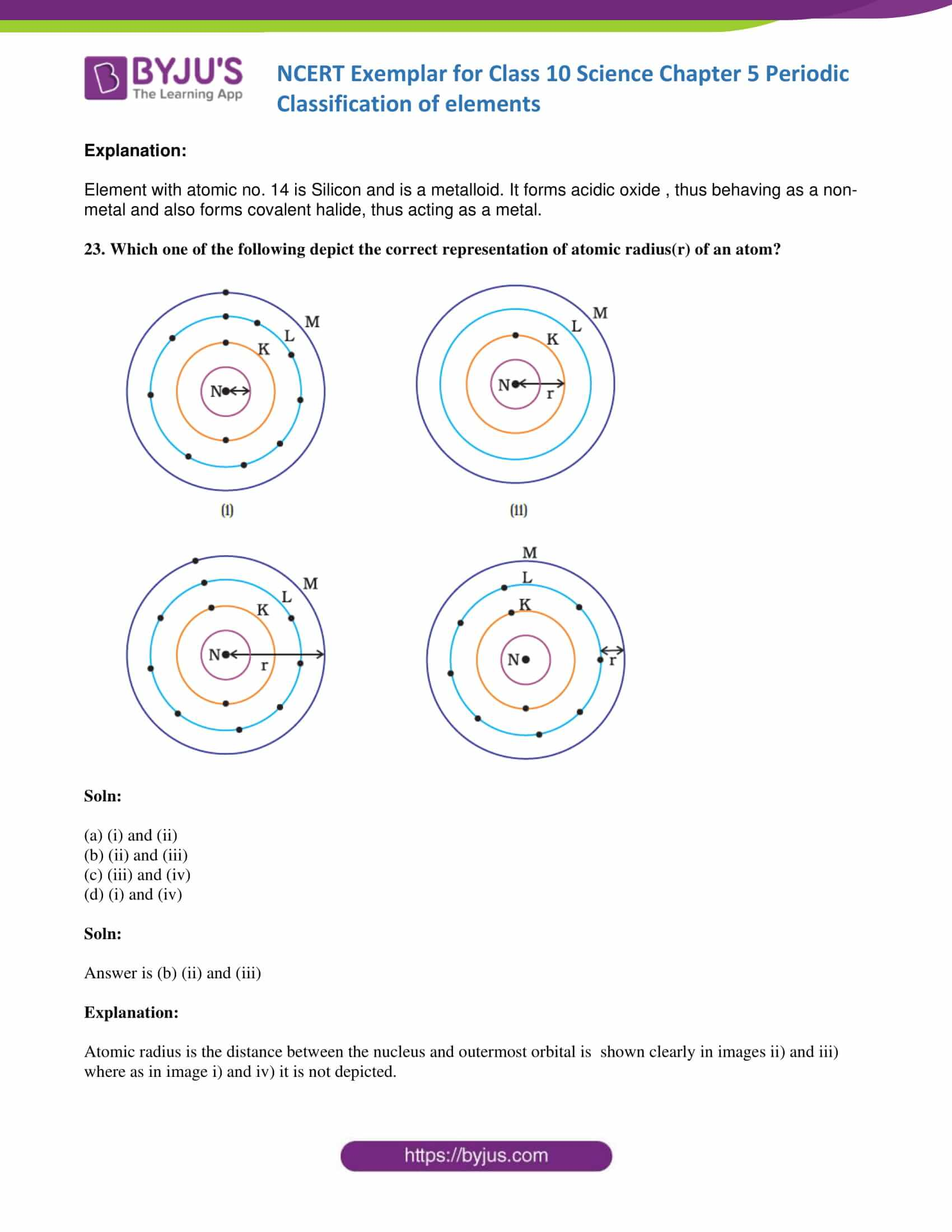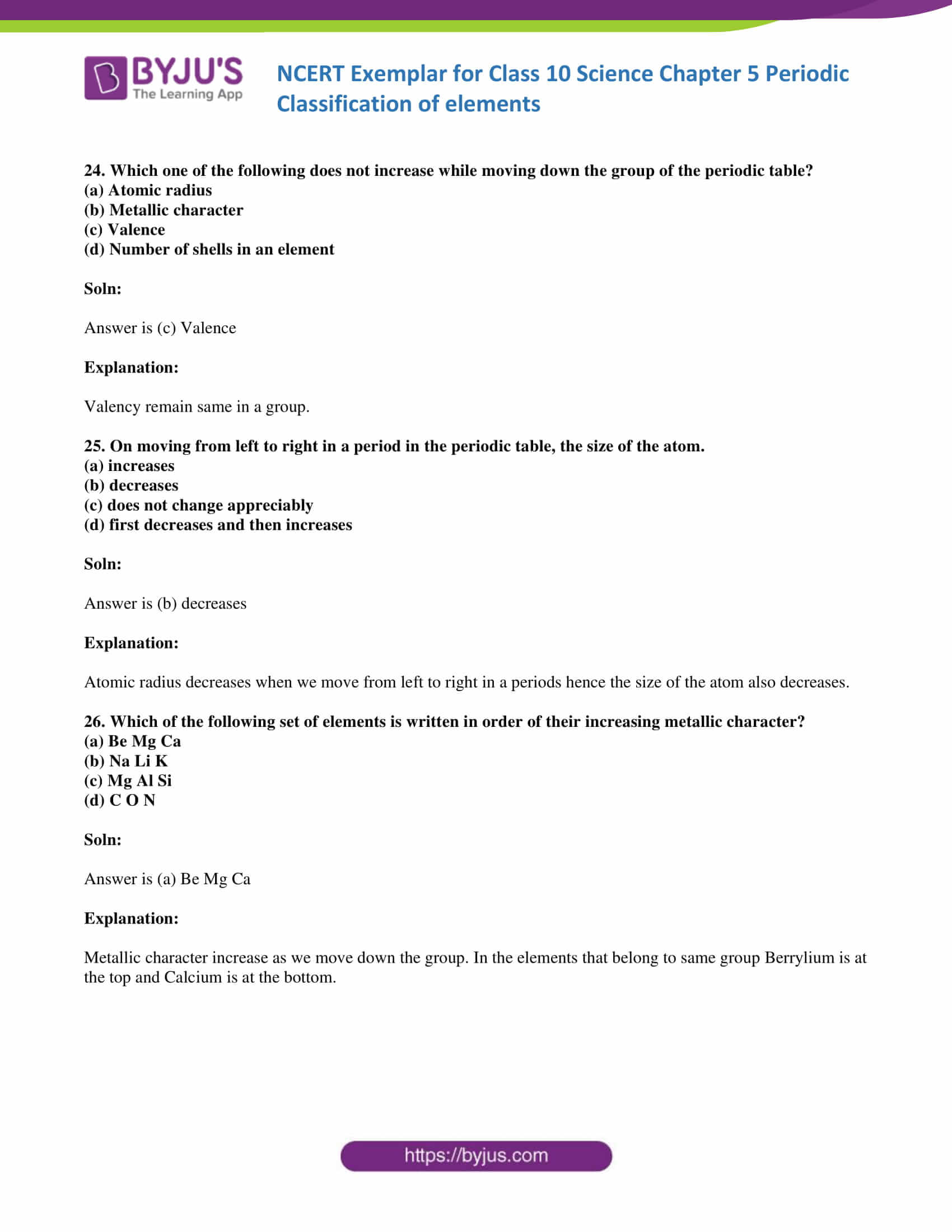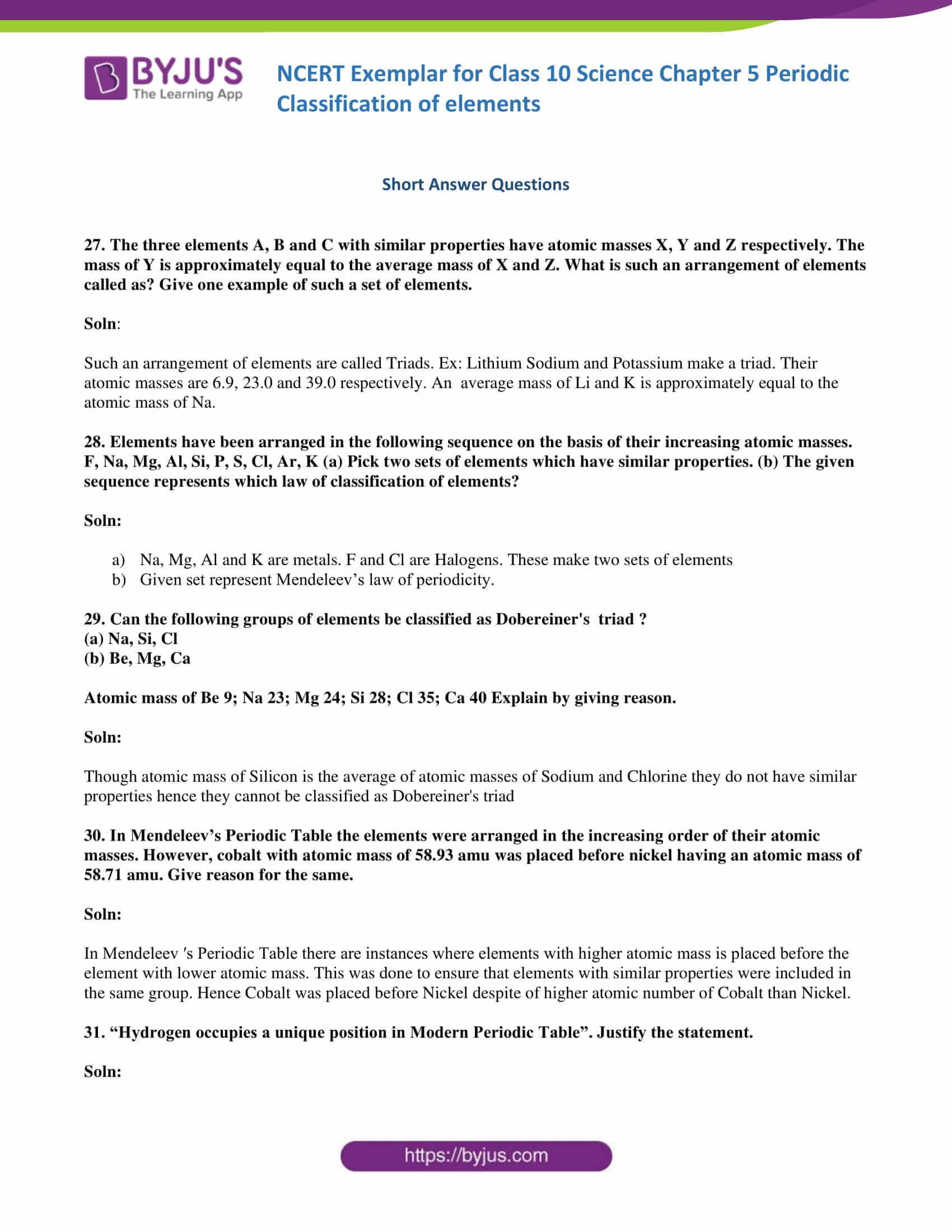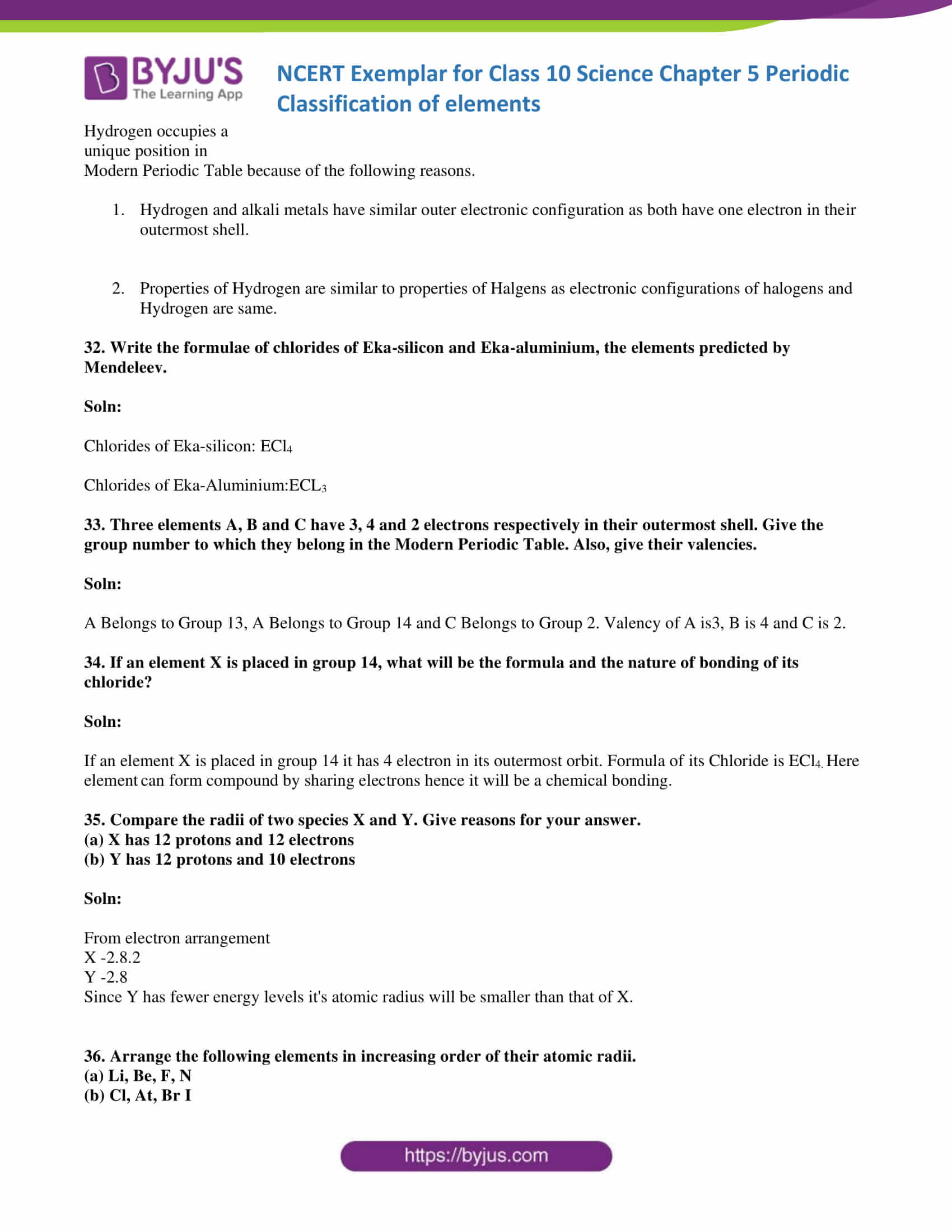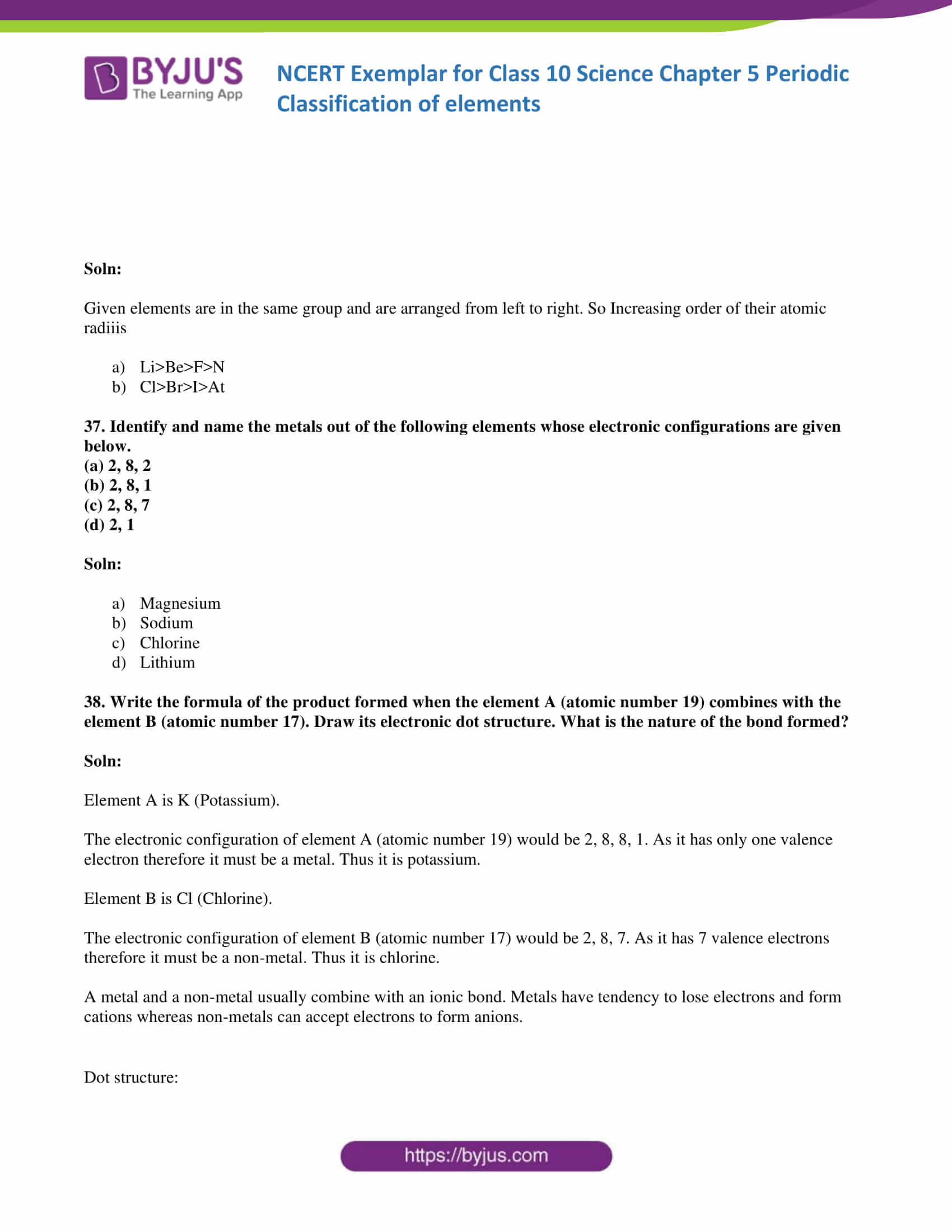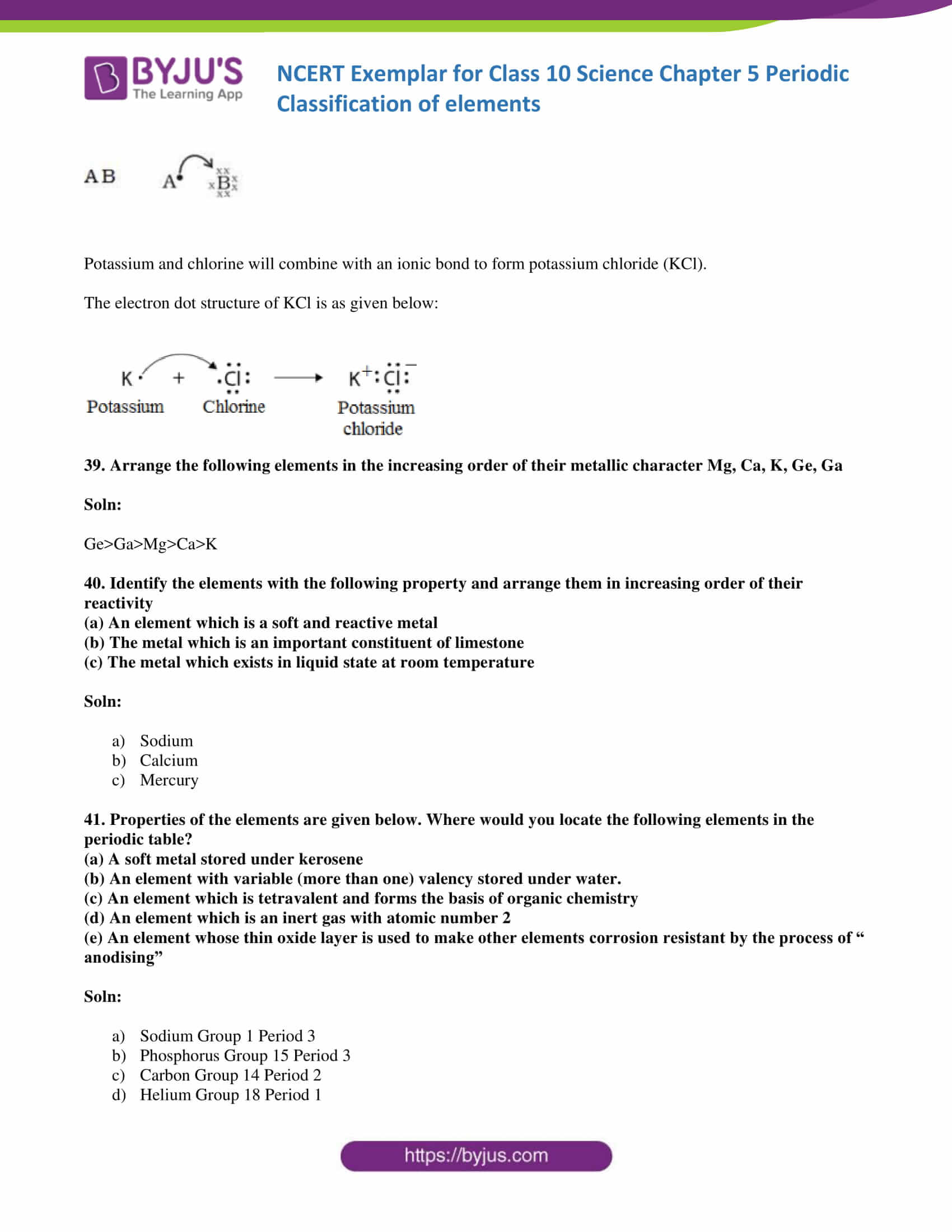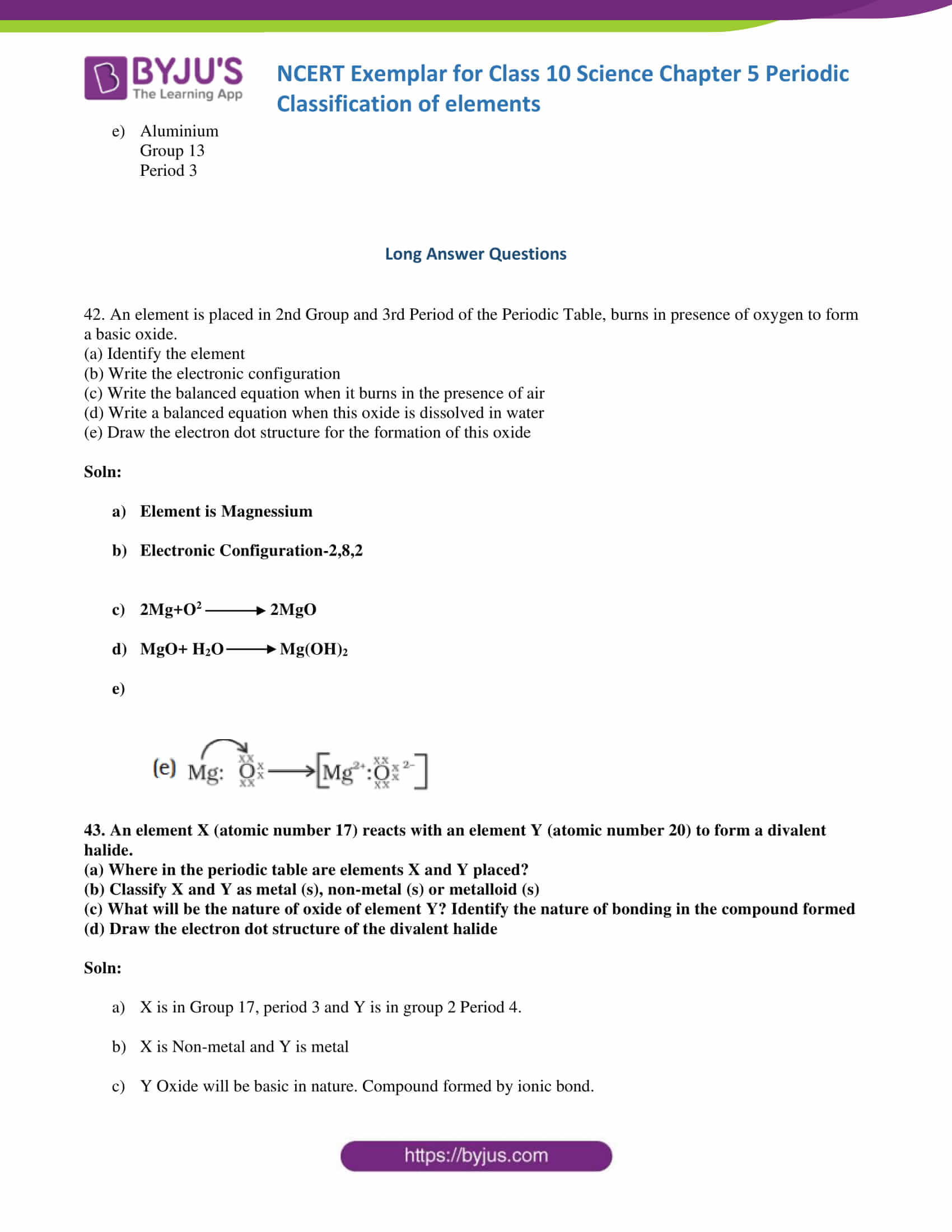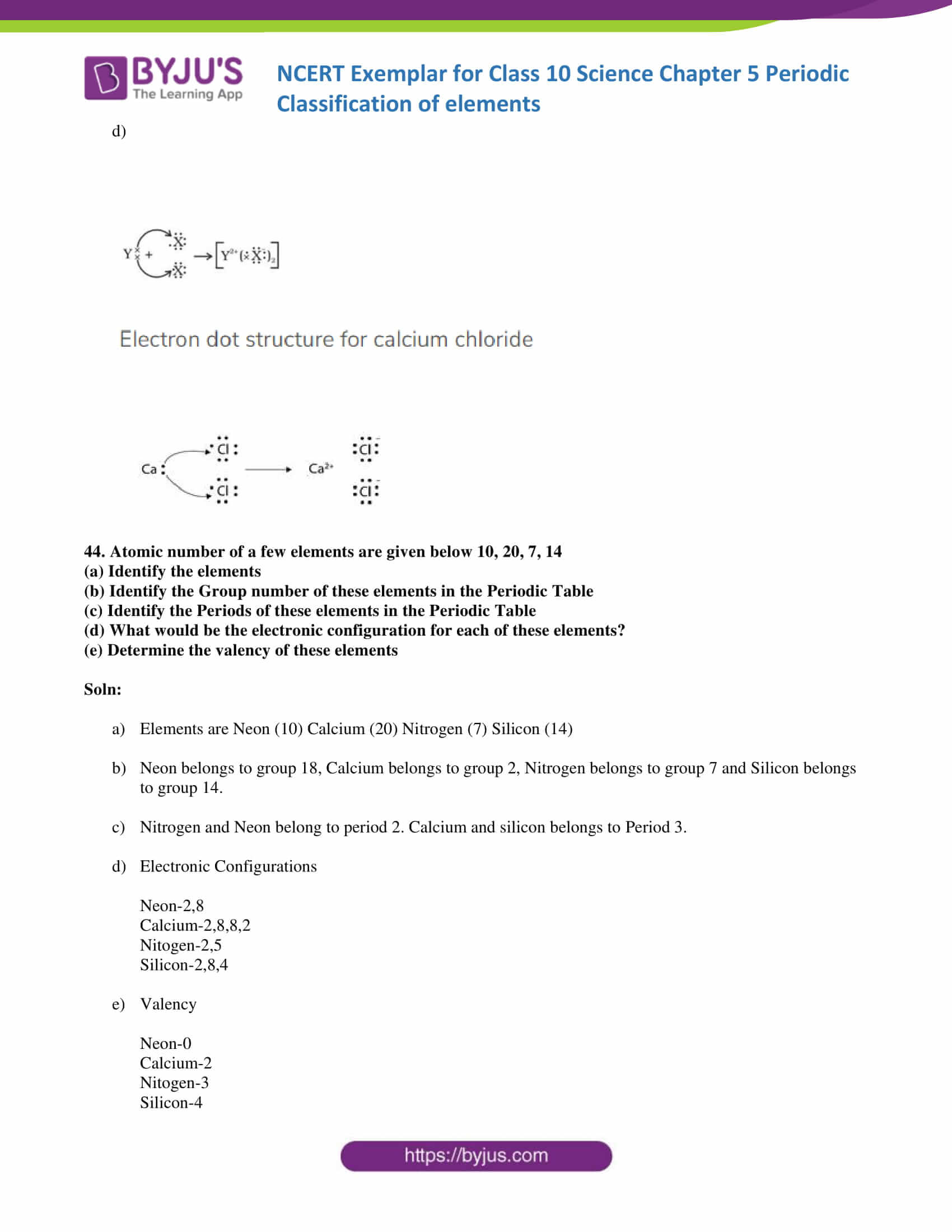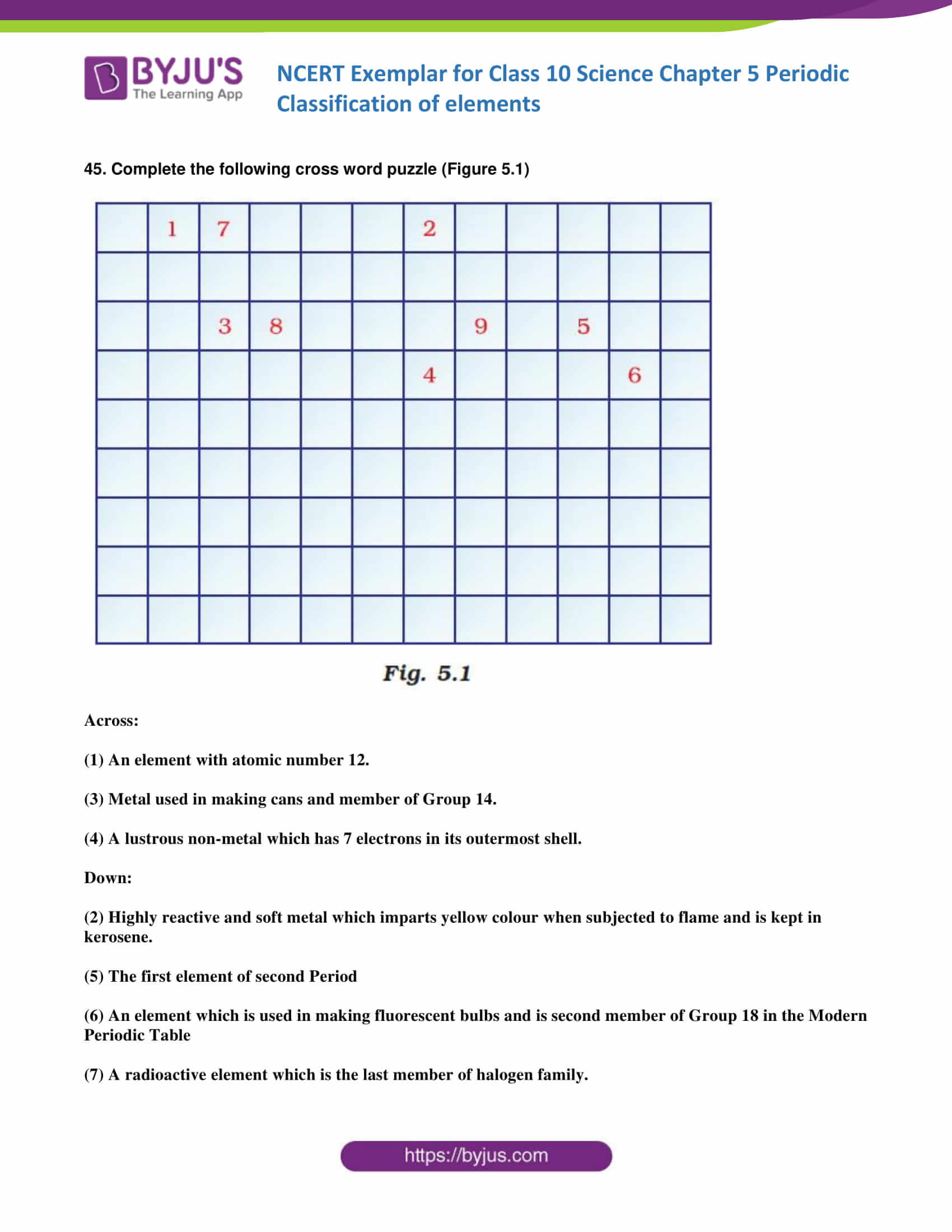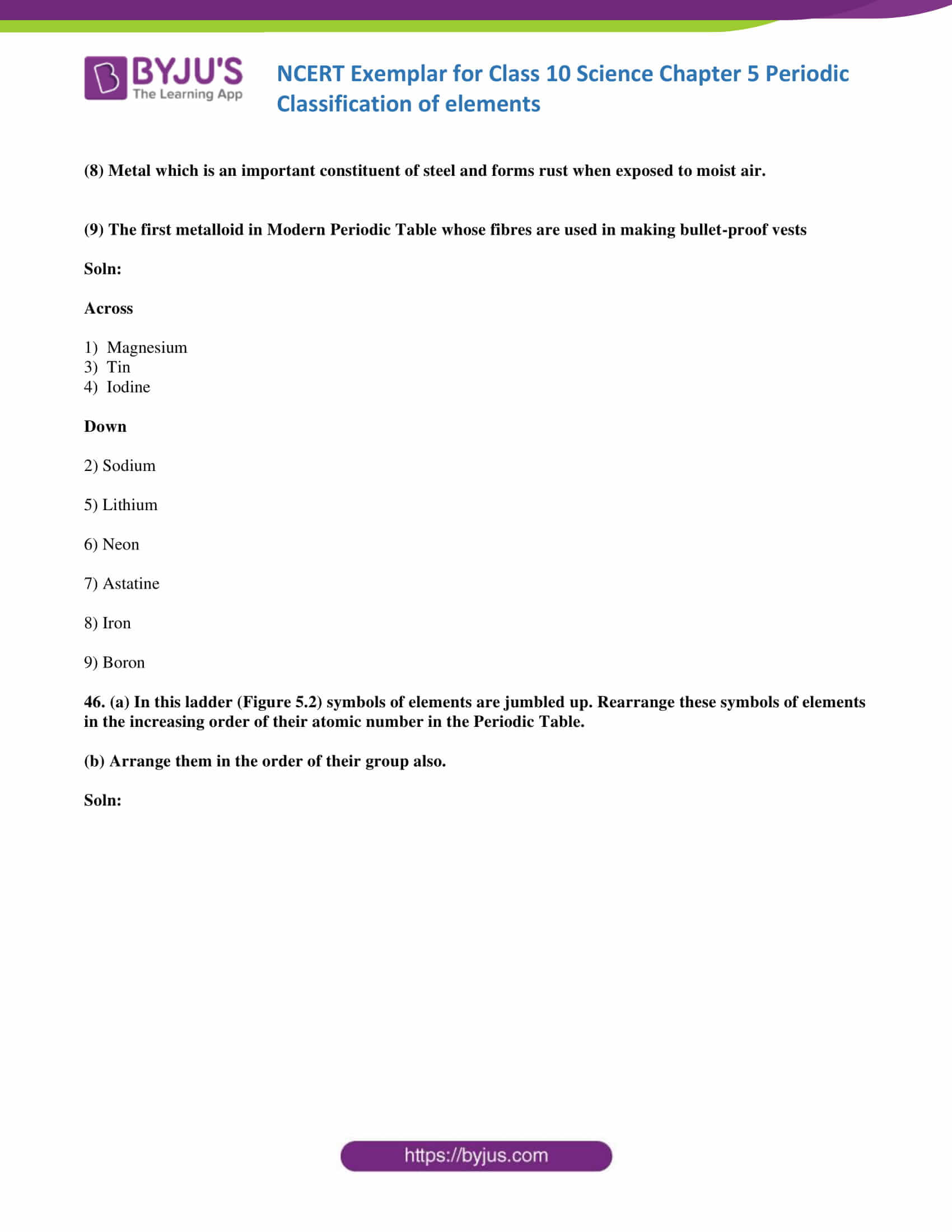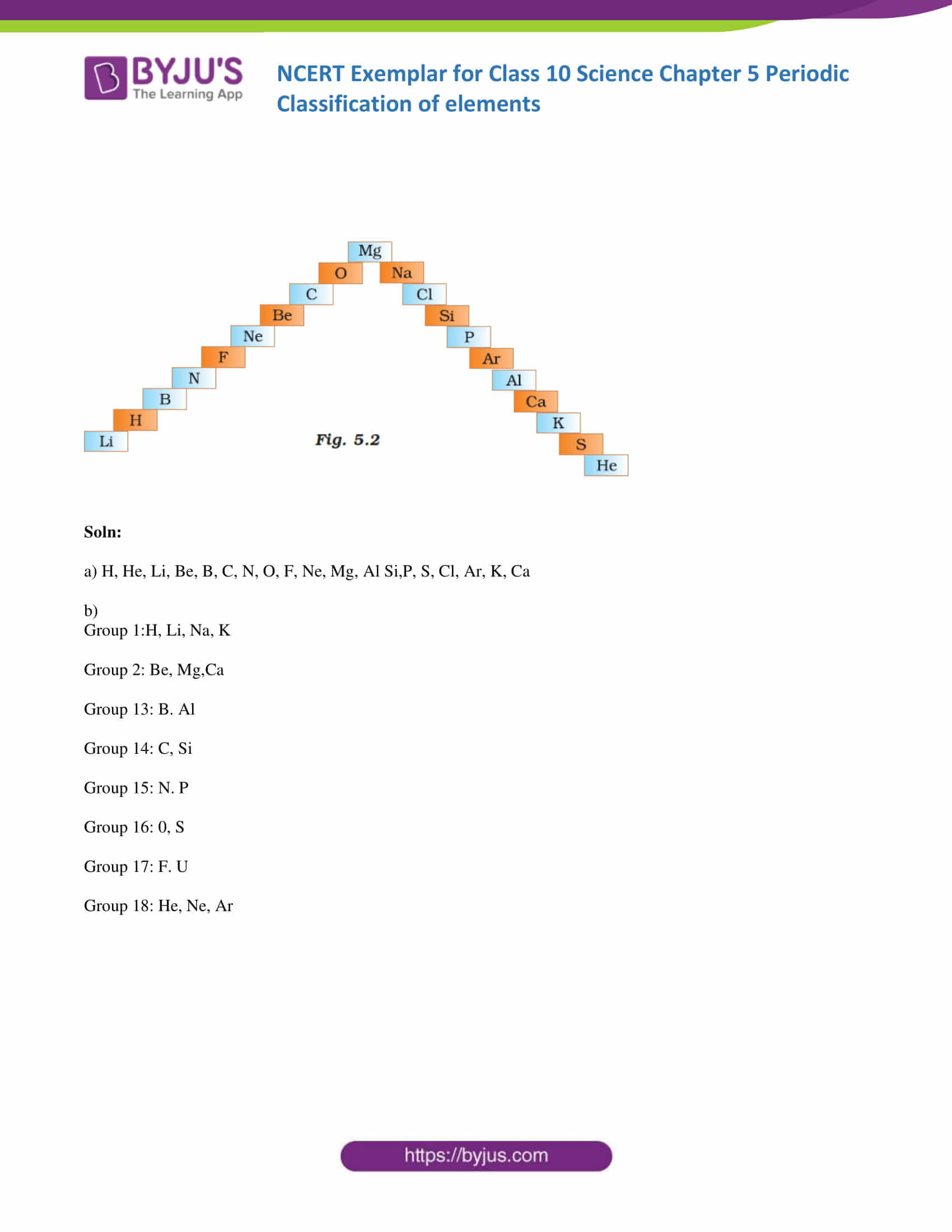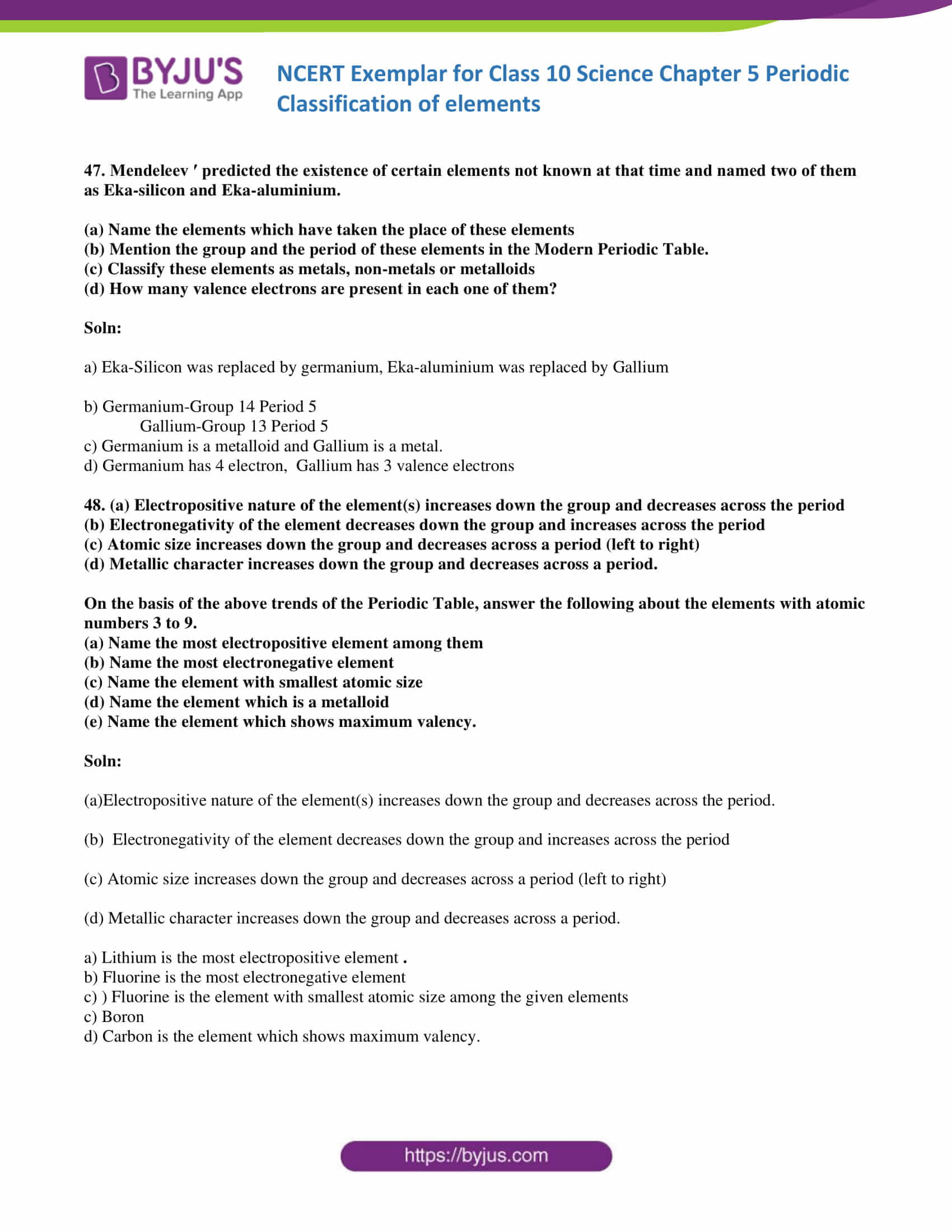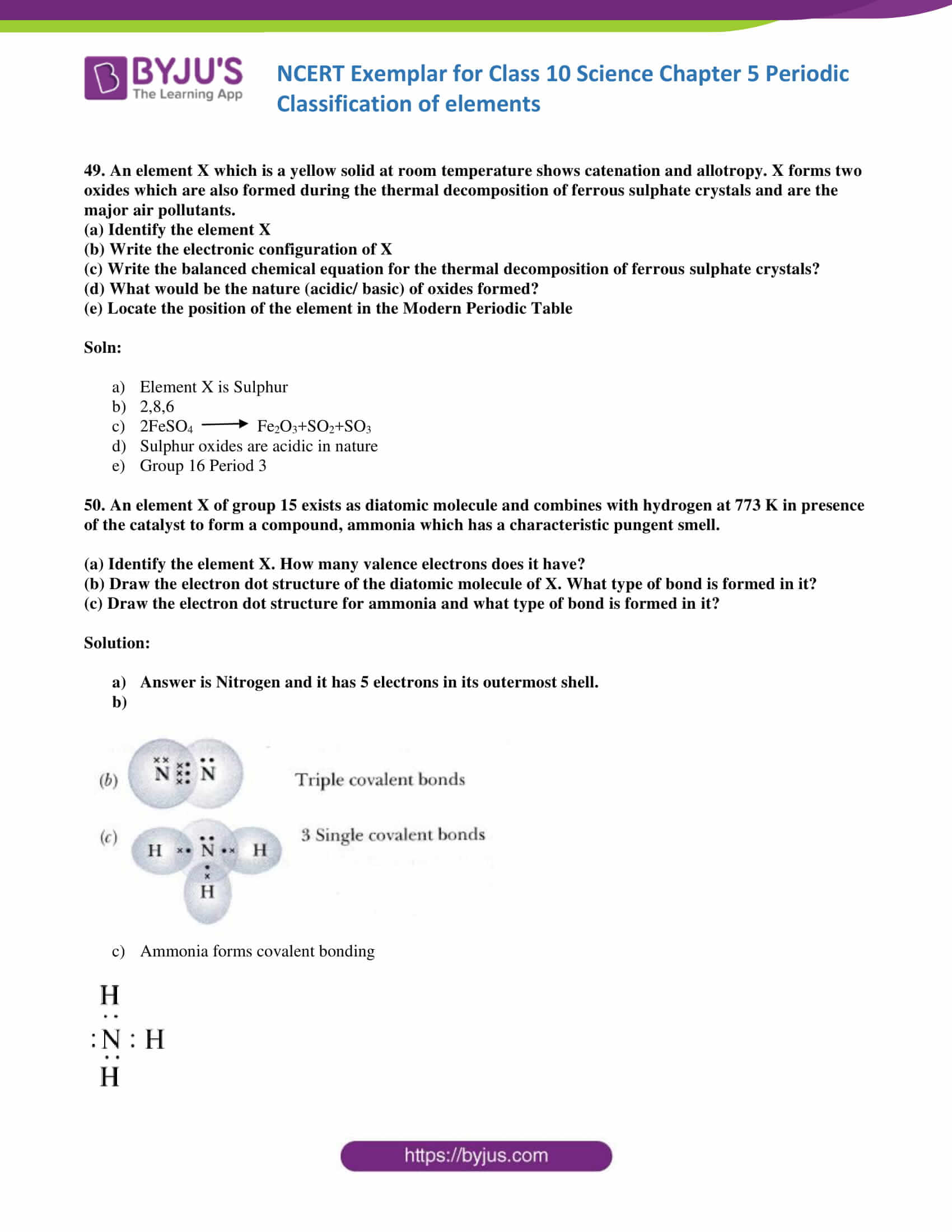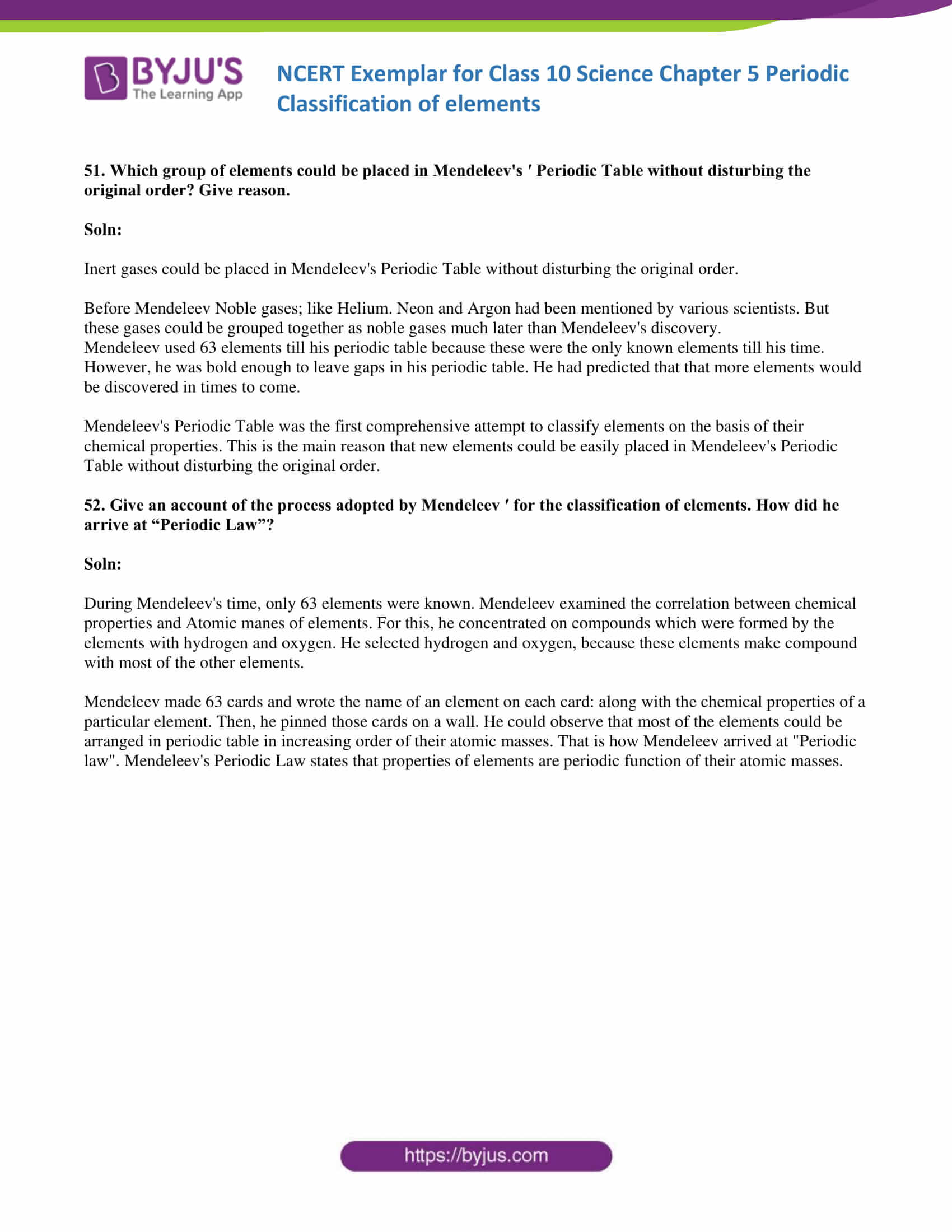### Multiple Choice Questions

1. Up to which element, the Law of Octaves was found to be applicable

(a) Oxygen

(b) Calcium

(c) Cobalt

(d) Potassium

Soln:

Explanation:

Newland’s law of octaves applied to the elements with atomic mass up to 40 da which comes up to Calcium. After calcium, every eighth element possess properties similar to that of the first.

2. According to Mendeleev’s ′ Periodic Law, the elements were arranged in the periodic table in the order of

(a) increasing atomic number

(b) decreasing atomic number

(c) increasing atomic masses

(d) decreasing atomic masses

Soln:

The answer is (c) increasing atomic masses

Explanation:

3. In Mendeleev ’s Periodic Table, gaps were left for the elements to be discovered later. Which of the following elements found a place in the periodic table later

(a) Germanium

(b) Chlorine

(c) Oxygen

(d) Silicon

Soln:

Explanation:

Mendeleev name unnamed elements as EKA- Boron EKA- Aluminium and EKA Silicon which were later replaced as Scandium, Gallium, and germanium respectively.

4. Which of the following statement (s) about the Modern Periodic Table are incorrect

(i) The elements in the Modern Periodic Table are arranged based on their decreasing atomic number

(ii) The elements in the Modern Periodic Table are arranged based on their increasing atomic masses

(iii) Isotopes are placed in adjoining group (s) in the Periodic Table

(iv) The elements in the Modern Periodic Table are arranged based on their increasing atomic number

(a) (i) only

(b) (i), (ii) and (iii)

(c) (i), (ii) and (iv)

(d) (iv) only

Soln:

The answer is (b) (i), (ii) and (iii)

Explanation:

Elements in the Modern Periodic Table are arranged based on their increasing atomic number hence option i) is wrong. In modern periodic table atomic mass is not a criteria hence option ii) is wrong. Isotopes are given the same position in periodic table hence option iii) is wrong.

5. Which of the following statements about the Modern Periodic Table is correct:

(a) It has 18 horizontal rows known as Periods

(b) It has 7 vertical columns known as Periods

(c) It has 18 vertical columns known as Groups

(d) It has 7 horizontal rows known as Groups

Soln:

The answer is (c) It has 18 vertical columns known as Groups

Explanation:

Modern periodic table have 18 groups and 7 periods. Columns are called groups and rows are called periods.

6. Which of the given elements A, B, C, D and E with atomic number 2, 3, 7, 10 and 30 respectively belong to the same period?

(a) A, B, C

(b) B, C, D

(c) A, D, E

(d) B, D, E

Soln:

The answer is (b) B, C, D

Explanation:

Element with atomic number belongs to the first period and element B, C and D belong to the second period.

7. The elements A, B, C, D and E have atomic number 9, 11, 17, 12 and 13 respectively. Which pair of elements belong to the same group?

(a) A and B

(b) B and D

(c) A and C

(d) D and E

Soln:

The answer is (c) A and C

Explanation:

A and C are fluorine and chlorine belongs to the same group.

8. Where would you locate the element with electronic configuration 2,8 in the Modern Periodic Table?

(a) Group 8

(b) Group 2

(c) Group 18

(d) Group 10

Soln:

The answer is (c) Group 18

Explanation:

Group 18 has its outermost shells completely filled hence element with electronic configuration belongs to group 18.

9. An element which is an essential constituent of all organic compounds belongs to

(a) group 1

(b) group 14

(c) group 15

(d) group 16

Soln:

The answer is b) group 14

Explanation:

Carbon is an essential constituent of all organic compounds which belongs to group 14.

10. Which of the following is the outermost shell for elements of period 2?

(a) K shell

(b) L shell

(c) M shell

(d) N shell

Soln:

The answer is (b) L shell

Explanation:

In period 18 there are two shells K and L

11. Which one of the following elements exhibit a maximum number of valence electrons?

(a) Na

(b) Al

(c) Si

(d) P

Soln:

Explanation:

Electronic configuration of Na, Al, Si and P are 2,8 1, 2,8,3,2,8,4, and 2,8,5 respectively. Valence electrons in Na, Al, Si and P are 1,3,4 and 5 Hence phosphorus has a maximum number of valence electrons.

12. Which of the following gives the correct increasing order of the atomic radii of O, F and N?

(a) O, F, N

(b) N, F, O
(c) O, N, F

(d) F, O, N

Soln:

The answer is (d) F, O, N

Explanation:

Atomic radius increase as move from left to right across a period. (N7), O(8) and F(10) are in increasing order of atomic numbers.

13. Which among the following elements has the largest atomic radii?

(a) Na

(b) Mg

(c) K

(d) Ca

Soln:

Soln:

K has the largest atomic radii because atomic radii decrease from left to right along a period. This is because of the increase in nuclear charge which tends to pull the electron closer to the nucleus and reduces the size of the atom. Hence K has the largest atomic radii.

14. Which of the following elements would lose an electron easily?

(a) Mg

(b) Na

(c) K

(d) Ca

Soln:

Explanation:

Na and K belong to the same group I and Magnesium and Calcium belongs to a group (II). Because K contains one electron in its outermost shell and the lowest ionisation energy, it can easily lose an electron.

15. Which of the following elements does not lose an electron easily?

(a) Na

(b) F

(c) Mg

(d) Al

Soln:

Sodium has 1, magnesium has 2 and Aluminium has 3 electrons in its outermost shell whereas Fluorine has 7 electrons in its outermost shell hence Fluorine does not lose electron easily.

16. Which of the following are the characteristics of isotopes of an element?

(i) Isotopes of an element have the same atomic masses

(ii) Isotopes of an element have the same atomic number

(iii) Isotopes of an element show the same physical properties

(iv) Isotopes of an element show the same chemical properties

(a) (i), (iii) and (iv)

(b) (ii), (iii) and (iv)

(c) (ii) and (iii)

(d) (ii) and (iv)

Soln:

The answer is (d) (ii) and (iv)

Explanation:

Elements with same atomic number but different atomic masses are known as isotopes. Isotopes same chemical properties but differ in their physical properties.

17. Arrange the following elements in the order of their decreasing metallic character Na, Si, Cl, Mg, Al

(a) Cl > Si >Al > Mg >Na

(b) Na >Mg >Al >Si > Cl

(c) Na > Al > Mg > Cl > Si

(d) Al > Na> Si > Ca> Mg

Soln:

The answer is (b) Na >Mg >Al >Si > Cl

Explanation:

Na has 1, magnesium has 2 , aluminium has 3 and Chlorine has 7 electrons in its valence shells. Hence Sodium shows maximum metallic characters followed by Magnessium, aluminium and chlorine shows non-metallic properties.

18. Arrange the following elements in the order of their increasing nonmetallic character Li, O, C, Be, F

(a) F < O < C < Be < Li

(b) Li < Be < C < O< F

(c) F < O < C < Be < Li

(d) F < O < Be < C < Li

Soln:

Li is on the left of in the 2nd period of modern periodic table followed by berrylium. Fluorine is at right next to Neon. Hence Fluorine shows maximum non-metallic characters followed by Oxygen, Berrylium, carbon and Lithium.

19. What type of oxide would Eka– aluminium form?

(a) EO3

(b) E3 O2

(c) E2 O3

(d) EO

Soln:

20. Three elements B, Si and Ge are

(a) metals

(b) non-metals

(c) metalloids

(d) metal, non-metal and metalloid respectively

Soln:

21. Which of the following elements will form an acidic oxide?

(a) An element with atomic number 7

(b) An element with atomic number 3

(c) An element with atomic number 12

(d) An element with atomic number 19

Soln:

The answer is (a) An element with atomic number 7

Explanation:

Element with atomic number 7 has electron configuration 2,5 which means it can gain 3 electrons and is an electronegative element. It should be a non-metal and non-metals will form acidic oxide.

22. The element with atomic number 14 is hard and forms acidic oxide and a covalent halide. To which of the following categories does the element belong?

(a) Metal

(b) Metalloid

(c) Non-metal

(d) Left-hand side element

Soln:

Explanation:

Element with atomic no. 14 is Silicon and is a metalloid. It forms an acidic oxide, thus behaving as a non- metal and also forms covalent halide, thus acting as a metal.

23. Which one of the following depicts the correct representation of the atomic radius(r) of an atom?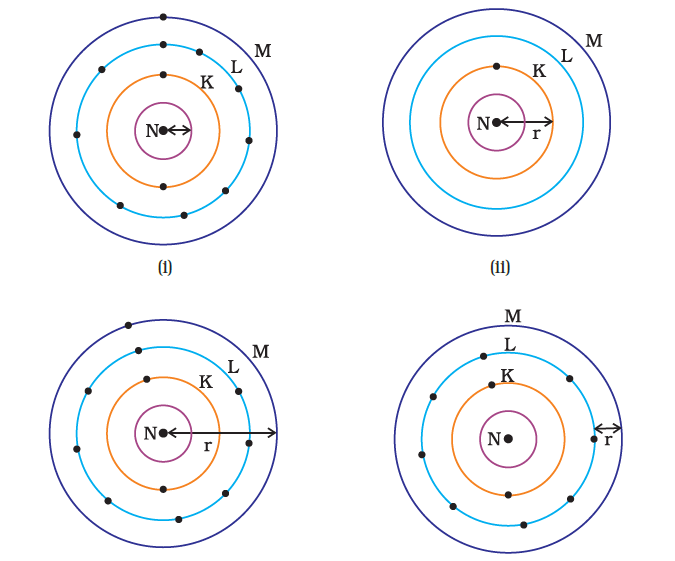Soln:

(a) (i) and (ii)

(b) (ii) and (iii)

(c) (iii) and (iv)

(d) (i) and (iv)

Soln:

The answer is (b) (ii) and (iii)

Explanation:

Atomic radius is the distance between the nucleus and outermost orbital is shown clearly in images ii) and iii) whereas in image i) and iv) it is not depicted.

24. Which one of the following does not increase while moving down the group of the periodic table?

(b) Metallic character

(c) Valence

(d) Number of shells in an element

Soln:

Explanation:

Valency remain the same in a group.

25. On moving from left to right in a period in the periodic table, the size of the atom.

(a) increases

(b) decreases

(c) does not change appreciably

(d) first decreases and then increases

Soln:

Explanation:

Atomic radius decreases when we move from left to right in a period hence the size of the atom also decreases.

26. Which of the following set of elements is written in order of their increasing metallic character?

(a) Be Mg Ca

(b) Na Li K

(c) Mg Al Si

(d) C O N

Soln:

The answer is (a) Be Mg Ca

Explanation:

Metallic character increase as we move down the group. In the elements that belong to the same group Berrylium is at the top and Calcium is at the bottom.

27. The three elements A, B and C with similar properties have atomic masses X, Y and Z respectively. The mass of Y is approximately equal to the average mass of X and Z. What is such an arrangement of elements called as? Give one example of such a set of elements.

Soln:

Such an arrangement of elements are called Triads. Ex: Lithium Sodium and Potassium make a triad. Their atomic masses are 6.9, 23.0 and 39.0 respectively. An average mass of Li and K is approximately equal to the atomic mass of Na.

28. Elements have been arranged in the following sequence on the basis of their increasing atomic masses. F, Na, Mg, Al, Si, P, S, Cl, Ar, K (a) Pick two sets of elements which have similar properties. (b) The given sequence represents which law of classification of elements?

Soln:

1. Na, Mg, Al and K are metals. F and Cl are Halogens. These make two sets of elements
2. Given set represent Mendeleev’s law of periodicity.

29. Can the following groups of elements be classified as Dobereiner’s triad?

(a) Na, Si, Cl

(b) Be, Mg, Ca

Atomic mass of Be 9; Na 23; Mg 24; Si 28; Cl 35; Ca 40 Explain by giving reason.

Soln:

Though atomic mass of Silicon is the average of atomic masses of Sodium and Chlorine they do not have similar properties hence they cannot be classified as Dobereiner’s triad

30. In Mendeleev’s Periodic Table the elements were arranged in the increasing order of their atomic masses. However, cobalt with an atomic mass of 58.93 amu was placed before nickel having an atomic mass of 58.71 amu. Give a reason for the same.

Soln:

In Mendeleev ′s Periodic Table there are instances where elements with higher atomic mass is placed before the element with lower atomic mass. This was done to ensure that elements with similar properties were included in the same group. Hence Cobalt was placed before Nickel despite of higher atomic number of Cobalt than Nickel.

31. “Hydrogen occupies a unique position in Modern Periodic Table”. Justify the statement.

Soln:

Hydrogen occupies a unique position in the Modern Periodic Table because of the following reasons.

1. Hydrogen and alkali metals have similar outer electronic configuration as both have one electron in their outermost shell.
2. Properties of Hydrogen are similar to properties of Halogens as electronic configurations of halogens and Hydrogen are same.

32. Write the formulae of chlorides of Eka-silicon and Eka-aluminium, the elements predicted by Mendeleev.

Soln:

Chlorides of Eka-silicon: ECl4

Chlorides of Eka-Aluminium: ECL3

33. Three elements A, B and C have 3, 4 and 2 electrons respectively in their outermost shell. Give the group number to which they belong in the Modern Periodic Table. Also, give their valencies.

Soln:

A Belongs to Group 13, B Belongs to Group 14 and C Belongs to Group 2. Valency of A is3, B is 4 and C is 2.

34. If an element X is placed in group 14, what will be the formula and the nature of bonding of its chloride?

Soln:

If an element X is placed in group 14 it has 4 electrons in its outermost orbit. Formula of its Chloride is ECl4. Here element can form compound by sharing electrons hence it will be a chemical bonding.

(a) X has 12 protons and 12 electrons

(b) Y has 12 protons and 10 electrons

Soln:

From electron arrangement

X -2.8.2

Y -2.8

Since Y has fewer energy levels it’s atomic radius will be smaller than that of X.

36. Arrange the following elements in increasing order of their atomic radii.

(a) Li, Be, F, N

(b) Cl, At, Br I

Soln:

Given elements are in the same group and are arranged from left to right. So Increasing order of their atomic radii

1. Li>Be>F>N
2. Cl>Br>I>At

37. Identify and name the metals out of the following elements whose electronic configurations are given below.

(a) 2, 8, 2

(b) 2, 8, 1

(c) 2, 8, 7

(d) 2, 1

Soln:

1. Magnesium
2. Sodium
3. Chlorine
4. Lithium

38. Write the formula of the product formed when the element A (atomic number 19) combines with the element B (atomic number 17). Draw its electronic dot structure. What is the nature of the bond formed?

Soln:

Element A is K (Potassium).

The electronic configuration of element A (atomic number 19) would be 2, 8, 8, 1. As it has only one valence electron therefore it must be a metal. Thus it is potassium.

Element B is Cl (Chlorine).

The electronic configuration of element B (atomic number 17) would be 2, 8, 7. As it has 7 valence electrons therefore it must be a non-metal. Thus it is chlorine.

A metal and a non-metal usually combine with an ionic bond. Metals tend to lose electrons and form cations whereas non-metals can accept electrons to form anions.

Dot structure: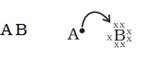Potassium and chlorine will combine with an ionic bond to form potassium chloride (KCl).

The electron dot structure of KCl is as given below: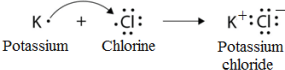39. Arrange the following elements in the increasing order of their metallic character Mg, Ca, K, Ge, Ga

Soln:

40. Identify the elements with the following property and arrange them in increasing order of their reactivity

(a) An element which is a soft and reactive metal

(b) The metal which is an important constituent of limestone

(c) The metal which exists in a liquid state at room temperature

Soln:

1. Sodium
2. Calcium
3. Mercury

41. Properties of the elements are given below. Where would you locate the following elements in the periodic table?

(a) A soft metal stored under kerosene

(b) An element with variable (more than one) valency stored underwater.

(c) An element which is tetravalent and forms the basis of organic chemistry

(d) An element which is an inert gas with atomic number 2

(e) An element whose thin oxide layer is used to make other elements corrosion-resistant by the process of “ anodising”

Soln:

1. Sodium Group 1 Period 3
2. Phosphorus Group 15 Period 3
3. Carbon Group 14 Period 2
4. Helium Group 18 Period 1
5. Aluminium Group 13 Period 3

42. An element is placed in 2nd Group and 3rd Period of the Periodic Table, burns in presence of oxygen to form a basic oxide.

(a) Identify the element

(b) Write the electronic configuration

(c) Write the balanced equation when it burns in the presence of air

(d) Write a balanced equation when this oxide is dissolved in water

(e) Draw the electron dot structure for the formation of this oxide

Soln:

(a) Element is Magnesium

(b) Electronic Configuration-2,8,2

(c) 2Mg+O2 2MgO

(d) MgO+ H2O Mg(OH)2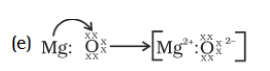43. An element X (atomic number 17) reacts with an element Y (atomic number 20) to form a divalent halide.

(a) Where in the periodic table are elements X and Y placed?

(b) Classify X and Y as metal (s), non-metal (s) or metalloid (s)

(c) What will be the nature of oxide of element Y? Identify the nature of bonding in the compound formed

(d) Draw the electron dot structure of the divalent halide

Soln:

(a)X is in Group 17, period 3 and Y is in group 2 Period 4.

(b)X is Non-metal and Y is metal

(c)Y Oxide will be basic in nature. Compound formed by ionic bond.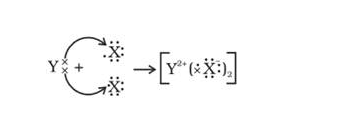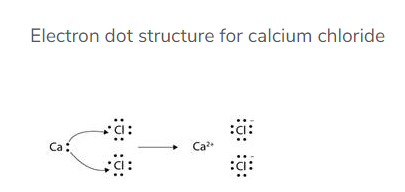44. Atomic number of a few elements are given below 10, 20, 7, 14

(a) Identify the elements

(b) Identify the Group number of these elements in the Periodic Table

(c) Identify the Periods of these elements in the Periodic Table

(d) What would be the electronic configuration for each of these elements?

(e) Determine the valency of these elements

Soln:

(a)Elements are Neon (10) Calcium (20) Nitrogen (7) Silicon (14)

(b)Neon belongs to group 18, Calcium belongs to group 2, Nitrogen belongs to group 7 and Silicon belongs to group 14.

(c)Nitrogen and Neon belong to period 2. Calcium and silicon belongs to Period 3.

(d)Electronic Configurations

Neon-2,8

Calcium-2,8,8,2

Nitrogen-2,5

Silicon-2,8,4

Valency of the elements

Neon-0

Calcium-2

Nitrogen-3

Silicon-4

45. Complete the following crossword puzzle (Figure 5.1)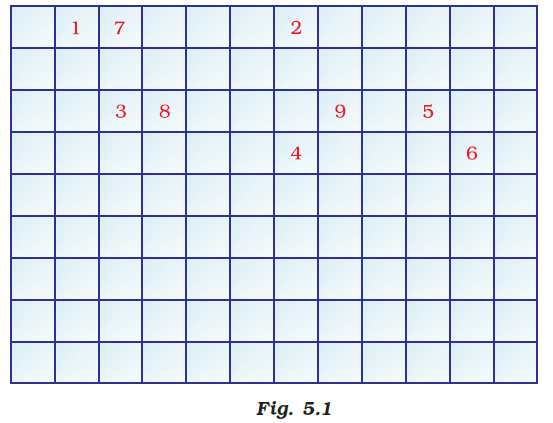Across:

(1) An element with atomic number 12.

(3) Metal used in making cans and member of Group 14.

(4) A lustrous non-metal which has 7 electrons in its outermost shell.

Down:

(2) Highly reactive and soft metal which imparts yellow colour when subjected to flame and is kept in kerosene.

(5) The first element of the second Period

(6) An element which is used in making fluorescent bulbs and is the second member of Group 18 in the Modern Periodic Table

(7) A radioactive element which is the last member of the halogen family.

(8) Metal which is an important constituent of steel and forms rust when exposed to moist air.

(9) The first metalloid in Modern Periodic Table whose fibres are used in making bullet-proof vests

Soln:

Across

1) Magnesium

3) Tin

4) Iodine

Down

2) Sodium

5) Lithium

6) Neon

7) Astatine

8) Iron

9) Boron

46. (a) In this ladder (Figure 5.2) symbols of elements are jumbled up. Rearrange these symbols of elements in the increasing order of their atomic number in the Periodic Table.

(b) Arrange them in the order of their group also.

Soln: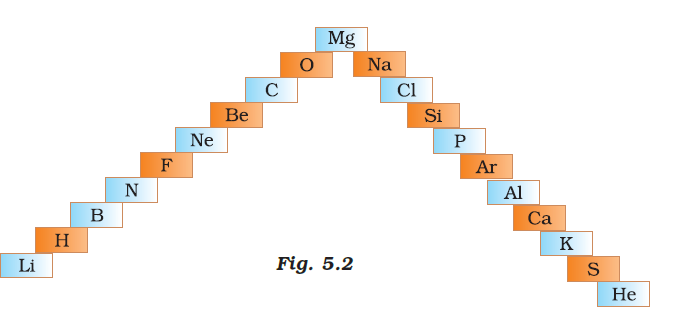Soln:

a) H, He, Li, Be, B, C, N, O, F, Ne, Mg, Al Si, P, S, Cl, Ar, K, Ca

b)

Group 1:H, Li, Na, K

Group 2: Be, Mg, Ca

Group 13: B. Al

Group 14: C, Si

Group 15: N. P

Group 16: 0, S

Group 17: F. U

Group 18: He, Ne, Ar

47. Mendeleev ′ predicted the existence of certain elements not known at that time and named two of them as Eka-silicon and Eka-aluminium.

(a) Name the elements which have taken the place of these elements

(b) Mention the group and the period of these elements in the Modern Periodic Table.

(c) Classify these elements as metals, non-metals or metalloids

(d) How many valence electrons are present in each one of them?

Soln:

a) Eka-Silicon was replaced by germanium, Eka-aluminium was replaced by Gallium

b) Germanium-Group 14 Period 4

Gallium-Group 13 Period 4

c) Germanium is a metalloid and Gallium is a metal.

d) Germanium has 4 electrons, Gallium has 3 valence electrons

48. (a) Electropositive nature of the element(s) increases down the group and decreases across the period

(b) Electronegativity of the element decreases down the group and increases across the period

(c) Atomic size increases down the group and decreases across a period (left to right)

(d) Metallic character increases down the group and decreases across a period.

Based on the above trends of the Periodic Table, answer the following about the elements with atomic numbers 3 to 9.

(a) Name the most electropositive element among them

(b) Name the most electronegative element

(c) Name the element with the smallest atomic size

(d) Name the element which is a metalloid

(e) Name the element which shows maximum valency.

Soln:

(a)Electropositive nature of the element(s) increases down the group and decreases across the period.

(b) Electronegativity of the element decreases down the group and increases across the period

(c) Atomic size increases down the group and decreases across a period (left to right)

(d) Metallic character increases down the group and decreases across a period.

a) Lithium is the most electropositive element.

b) Fluorine is the most electronegative element

c) ) Fluorine is the element with smallest atomic size among the given elements

c) Boron

d) Carbon is the element which shows maximum valency.

49. An element X which is a yellow solid at room temperature shows catenation and allotropy. X forms two oxides which are also formed during the thermal decomposition of ferrous sulphate crystals and are the major air pollutants.

(a) Identify the element X

(b) Write the electronic configuration of X

(c) Write the balanced chemical equation for the thermal decomposition of ferrous sulphate crystals?

(d) What would be the nature (acidic/ basic) of oxides formed?

(e) Locate the position of the element in the Modern Periodic Table

Soln:

1. Element X is Sulphur
2. 2,8,6
3. 2FeSO4 Fe2O3+SO2+SO3
4. Sulphur oxides are acidic in nature
5. Group 16 Period 3

50. An element X of group 15 exists as a diatomic molecule and combines with hydrogen at 773 K in presence of the catalyst to form a compound, ammonia which has a characteristic pungent smell.

(a) Identify the element X. How many valence electrons does it have?

(b) Draw the electron dot structure of the diatomic molecule of X. What type of bond is formed in it?

(c) Draw the electron dot structure for ammonia and what type of bond is formed in it?

Solution:

1. Answer is Nitrogen and it has 5 electrons in its outermost shell.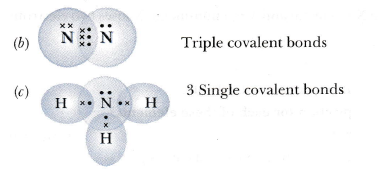1. Ammonia forms covalent bonding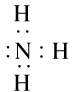51. Which group of elements could be placed in Mendeleev’s ′ Periodic Table without disturbing the original order? Give reason.

Soln:

Inert gases could be placed in Mendeleev’s Periodic Table without disturbing the original order.

Before Mendeleev Noble gases; like Helium. Neon and Argon had been mentioned by various scientists. But these gases could be grouped as noble gases much later than Mendeleev’s discovery.

Mendeleev used 63 elements till his periodic table because these were the only known elements till his time. However, he was bold enough to leave gaps in his periodic table. He had predicted that more elements would be discovered in times to come.

Mendeleev’s Periodic Table was the first comprehensive attempt to classify elements based on their chemical properties. This is the main reason that new elements could be easily placed in Mendeleev’s Periodic Table without disturbing the original order.

52. Give an account of the process adopted by Mendeleev ′ for the classification of elements. How did he arrive at “Periodic Law”?

Soln:

During Mendeleev’s time, only 63 elements were known. Mendeleev examined the correlation between chemical properties and Atomic manes of elements. For this, he concentrated on compounds which were formed by the elements with hydrogen and oxygen. He selected hydrogen and oxygen, because these elements make a compound with most of the other elements.

Mendeleev made 63 cards and wrote the name of an element on each card: along with the chemical properties of a particular element. Then, he pinned those cards on a wall. He could observe that most of the elements could be arranged in the periodic table in increasing order of their atomic masses. That is how Mendeleev arrived at “Periodic law”. Mendeleev’s Periodic Law states that properties of elements are periodic function of their atomic masses.

### Class 10 Periodic Classification of Elements NCERT Exemplar

This NCERT Exemplar has questions on the periodic table with answers, questions on the position of elements in the modern periodic table, periodic classification in NEET questions, important electronic configurations, numerical problems, MCQs, match the following and questions from sample papers and previous year question papers. Periodic Classification of Elements is a crucial chapter for the Class 10 CBSE board examination. This is also important for competitive examinations, as many questions are usually asked on the topics included in the chapter.

Class 10 Periodic Classification of Elements Important Concepts

1. Making Order Out of Chaos – Early Attempts at the Classification of Elements

1.2 Newlands’ Law of Octaves

2. Making Order Out of Chaos – Mendeleev’s Periodic Table

2.1 Achievements of Mendeleev’s Periodic Table

2.2 Limitations of Mendeleev’s Classification

3. Making Order Out of Chaos – The Modern Periodic

3.1 Position of Elements in the Modern Periodic

3.2 Trends in the Modern Periodic Table

To get acquainted with the concepts involved in this chapter, students are advised to solve sample papers and NCERT exemplars provided by BYJU’S. Students can download BYJU’S – The Learning App to get access to all study materials provided by us.

## Frequently Asked Questions on NCERT Exemplar Solutions for Class 10 Science Chapter 5

Q1

### What topics and sub-topics are covered in Chapter 5 of NCERT Exemplar Solutions for Class 10 Science?

The topics and sub-topics covered in Chapter 5 of NCERT Exemplar Solutions for Class 10 Science are
1. Making Order Out of Chaos – Early Attempts at the Classification of Elements
1.2 Newlands’ Law of Octaves
2. Making Order Out of Chaos – Mendeleev’s Periodic Table
2.1 Achievements of Mendeleev’s Periodic Table
2.2 Limitations of Mendeleev’s Classification
3. Making Order Out of Chaos – The Modern Periodic
3.1 Position of Elements in the Modern Periodic
3.2 Trends in the Modern Periodic Table
Q2

### What are the demerits of Mendeleev’s periodic table in the NCERT Exemplar Solutions for Class 10 Science Chapter 5?

Demerits of the Mendeleev periodic table are
1. He was unable to locate hydrogen in the periodic table.
2. Increase in atomic mass was not regular while moving from one element to another. Hence, the number of elements yet to be discovered was not predictable.
3. Later on, isotopes of elements were found that violated Mendeleev’s periodic law.
Q3

### How are NCERT Exemplar Solutions for Class 10 Science Chapter 5 designed at BYJU’S?

NCERT Solutions designed at BYJU’S are highly accurate and apt as per the latest CBSE guidelines. These solutions are the best reference materials which can be used by students for their exam preparation. They can build their conceptual understanding of fundamental topics which are important for the exam. Each concept also contains examples and pictorial representation to improve visual learning among students. These solutions improve smart learning and efficient answering skills among students, which results in a better academic score.